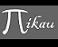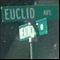Discussion about math, puzzles, games and fun.   Useful symbols: ÷ × ½ √ ∞ ≠ ≤ ≥ ≈ ⇒ ± ∈ Δ θ ∴ ∑ ∫  π  -¹ ² ³ °

You are not logged in.

## #1 2006-05-08 07:27:09

mikau
MemberRegistered: 2005-08-22
Posts: 1,504

### function effeciency: rotation

Some of you may recall my 3d engine thread. I've used this simple engine to create a simple vector game demo, however I'm begining to notice some slow down when too many vectors are present, and I believe its from the dozens of rotations being made. So I'm trying to find a way to make my rotate function more effecient.

Now the way the rotation function works, is it takes the x,y coordinates of a point, the x,y coordinates of a center point, and an angle, it then rotates the point around the center point and reassigns its x, y coordinates. I do this by basicly converting to polar form, which calls a polarAngle function (which uses arctan and a few if statements) and a distance function (thew distance formula) after the point has been converted into polarform, the polar angle is increased by the angle of rotation and they are then converted back to rectangular form. So for each rotation, arctan is called once for converting to polarform, sqrt is called in the distance formula, and sine and cosine are called when converting back to rectangular form. Those are some pretty time consuming functions aren't they?

I'm trying to find a more efficent way of doing it. One way I thought of is using trig identities for sin (a + b) and cos (a + b). Basicly a would be its polarAngle and b the angle of rotation, but sin a and sin b I can find using their rectangular coordinates and the distance formula. That avoids calling arctan. So all we need is the sin and cosine of the angle of rotation. Calling this is unavoidable but often times I have to rotate a group of points by the same angle, perhaps I could save the cosine and sine of the angle as a global variable, then in the next calling of the function, it checks to see if its the same angle as the previous calling of the function, if it is then the already calculated sine and cosine are called and you don't have to call the trig functions again.

Ok, so here's what it should look like, first remember sin ( a + b) = sin a cos b + cos a sin b and  cos (a + b) = cos a cos b - sin a sin b

rotateXY( vector &cent, vector &point, float &angle)
{

float the_distance = distance( cent.x, cent.y, point.x, point.y );

sin_a = (point.y - cent.y)/the_distance;
cos_a  = (point.x - cent.x)/the_distance;

if ( angle != prev_angle )        // prev_angle is a global variable
{

prev_cos = cos ( angle );         // prev_cos is a global variable
prev_sin = sin ( angle ) ;         // prev_sin is a global variable

prev_angle = angle;

}

point.x = cent.x + the_distance * ( cos_a * prev_cos - sin_a * prev_sin );

point.y = cent.y + the_distance * ( sin_a * prev_cos + cos_a * prev_sin );

}

}

What I'm wondering is I few in a few extra parts, like the if statement to check to see if its the same angle, and then reassigning the prev_cos, prev_sin and prev_angle variables. Could throwing these extra variables and conditions in actually do more harm then good? Also converting back to rectangular form we use a few multiplications instead of just dist * cos or sin.

Can anyone think of a better way to do this or improvements I could make to the code?

Last edited by mikau (2006-05-08 07:40:55)

A logarithm is just a misspelled algorithm.

Offline

## #2 2006-05-08 09:56:21

MathsIsFun
AdministratorRegistered: 2005-01-21
Posts: 7,691

### Re: function effeciency: rotation

I have an idea for you, something I use myself.

Create a function (I call mine "here"): each time you call this function it adds the time and a given name to arrays. At the end of your program, take those arrays and sum up the time differences vs names and print them out. This will show you where most time is being spent!

So you would throw in several calls to here in your code like this:

...
here("r1");
sin_a = (point.y - cent.y)/the_distance;
cos_a  = (point.x - cent.x)/the_distance;
here("r2");
if ( angle != prev_angle )        // prev_angle is a global variable
{
here("r3");
prev_cos = cos ( angle );         // prev_cos is a global variable
prev_sin = sin ( angle ) ;         // prev_sin is a global variable
...

And you would aim for an output showing "from", "to", count and total time like this

r1 r2 1500 0.567
r2 r3 300 0.123

It may take a little while to get your "here" function to work, but it has wonderful uses everywhere.

Sorry, but I actually don't have a C version I can show you, as mine was in Visual Basic when I was doing some time-intensive Database work.

(Note: I actually build in the "sum the differences and output result" into the here() function, triggered by calling "here('end')")

"The physicists defer only to mathematicians, and the mathematicians defer only to God ..."  - Leon M. Lederman

Offline

## #3 2006-05-08 10:15:36

mikau
MemberRegistered: 2005-08-22
Posts: 1,504

### Re: function effeciency: rotation

Interesting, thanks for the tip, mathsisfun!

Another less eleqent way I'm going to try is looping it like 1,000,000,000 times and use a stop watch to test both methods. If the new method helped there should be a major difference.

A logarithm is just a misspelled algorithm.

Offline

## #4 2006-05-08 10:23:53

MathsIsFun
AdministratorRegistered: 2005-01-21
Posts: 7,691

### Re: function effeciency: rotation

I found the VB Code - it actually places the result into a database table (HereSet) but you could put it into another array, then print it out after:

``````Sub Here(HereLocn As String)

Static Count As Integer
Static Locn(20000) As String
Static When(20000) As Double

Locn(Count) = HereLocn
When(Count) = Timer
Count = Count+1;

If HereLocn = "End" Then
Dim i As Integer, Elapsed As Double, LocnPrev As String

For i = 0 To Count - 1
If i <> 0 Then
Elapsed = When(i) - When(i - 1)
LocnPrev = Locn(i - 1)
Else
Elapsed = 0
LocnPrev = "Start"
End If

HereSet.AddNew
HereSet("Locn") = Locn(i)
HereSet("LocnPrev") = LocnPrev
HereSet("When") = When(i) - When(0)
HereSet("Elapsed") = Elapsed
HereSet.Update
Next
End If

End Sub``````

Not only does it take the guesswork out of where your bottlenecks are, it gives you a picture of where your code is spending most time and what areas NOT to worry about (ie you may have some really inefficient code, but if it's total execution time is only 5 milliseconds then don't waste your time improving it)

"The physicists defer only to mathematicians, and the mathematicians defer only to God ..."  - Leon M. Lederman

Offline

## #5 2006-05-08 14:28:13

mikau
MemberRegistered: 2005-08-22
Posts: 1,504

### Re: function effeciency: rotation

Well I tried looping the new and old function ten million times, I changed the angle every 5 loops because in my program I'm usually rotating groups of vectors by the same angle.

Results: looping ten million times the old method took about 6 seconds, the new took about 3. Not bad at all... and I actually think it will be more effecient in my program as the angle changes less.

Also I started this thread for finding math functions that are more effecient.

For instance is it quicker use:

y = sin θ;
x = cos θ;

or

y = sin θ
x = sqrt ( 1 - y^2);

???

Last edited by mikau (2006-05-09 06:54:40)

A logarithm is just a misspelled algorithm.

Offline

## #6 2006-05-09 05:33:16

ryos
MemberRegistered: 2005-08-04
Posts: 394

### Re: function effeciency: rotation

You could try a "quick and dirty" implementation of trig functions; namely, store a hash of precomputed values and interpolate between them as needed for intermediate angles. I know that many calculators do it this way. C's function may also work this way, so you may not actually gain anything by trying this (maybe you could find documentation on it?). I would hope, though, that they would have optimized the API function for accuracy more than for speed.

El que pega primero pega dos veces.

Offline

## #7 2006-05-09 06:51:48

Ricky
ModeratorRegistered: 2005-12-04
Posts: 3,791

### Re: function effeciency: rotation

Processors such as pentiums have support for trip functions on the hardware level.  So a hash table is actually slower than using cos and sin in C/C++.

But with things like integrated circuits (cheap processors, like ones in microwaves), you'd be right.

"In the real world, this would be a problem.  But in mathematics, we can just define a place where this problem doesn't exist.  So we'll go ahead and do that now..."

Offline

## #8 2006-05-09 06:53:46

John E. Franklin
MemberRegistered: 2005-08-29
Posts: 3,588

### Re: function effeciency: rotation

I thought they added trig functions to the math co-processor back with the 80386...  I might be wrong though.  If the pentium has the trig on the chip, the way you compile may allow it to be used??  Just a guess.  Read the compiler manual for math stuff.

igloo myrtilles fourmis

Offline

## #9 2006-05-09 06:59:49

Ricky
ModeratorRegistered: 2005-12-04
Posts: 3,791

### Re: function effeciency: rotation

Any decent compiler will use the specific chip trig functions for their implementation of the standard library math functions.  G++, VC++ (microsoft), borland all do.

"In the real world, this would be a problem.  But in mathematics, we can just define a place where this problem doesn't exist.  So we'll go ahead and do that now..."

Offline

## #10 2006-05-09 10:10:33

mikau
MemberRegistered: 2005-08-22
Posts: 1,504

### Re: function effeciency: rotation

Well I tried using:

y = sin θ;
x = cos θ;

and comparing it to:

y = sin θ
x = sqrt ( 1 - y^2);

The first took about 9 seconds with one hundred million loops, the second took 4 seconds. It seems it is faster, however sqrt ( 1 - y^2) always returns a positive value. Geez I never stopped to think about that untill now! Thats wierd. You use that identity for substitutions lots of times in trig, and in calculus, its not even always a valid subsitution! Why does it work then I wonder...

How irritating...

Last edited by mikau (2006-05-09 10:35:45)

A logarithm is just a misspelled algorithm.

Offline

## #11 2006-05-09 10:52:23

mikau
MemberRegistered: 2005-08-22
Posts: 1,504

### Re: function effeciency: rotation

Oh and btw I implemented the rotation function I mentioned and the slowdown has dissapeared.  :-) I also have a few extra simplifications I'm going to make which should speed things up.

A logarithm is just a misspelled algorithm.

Offline

## Board footer

Powered by FluxBB